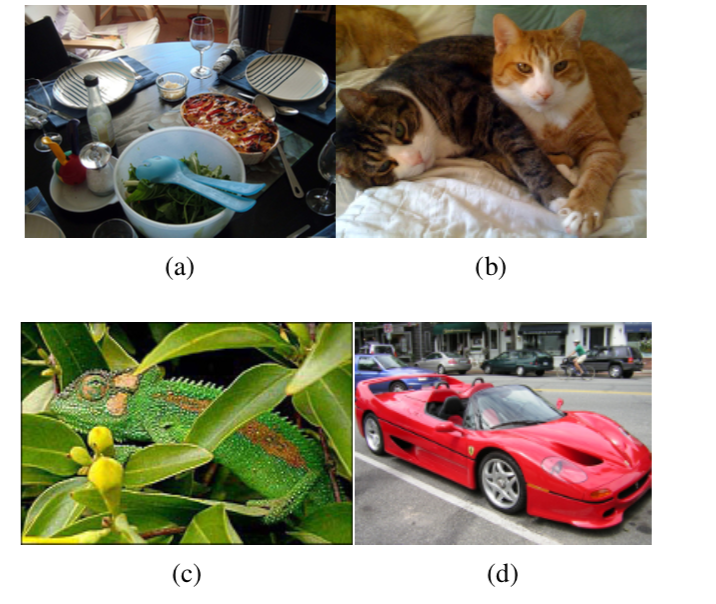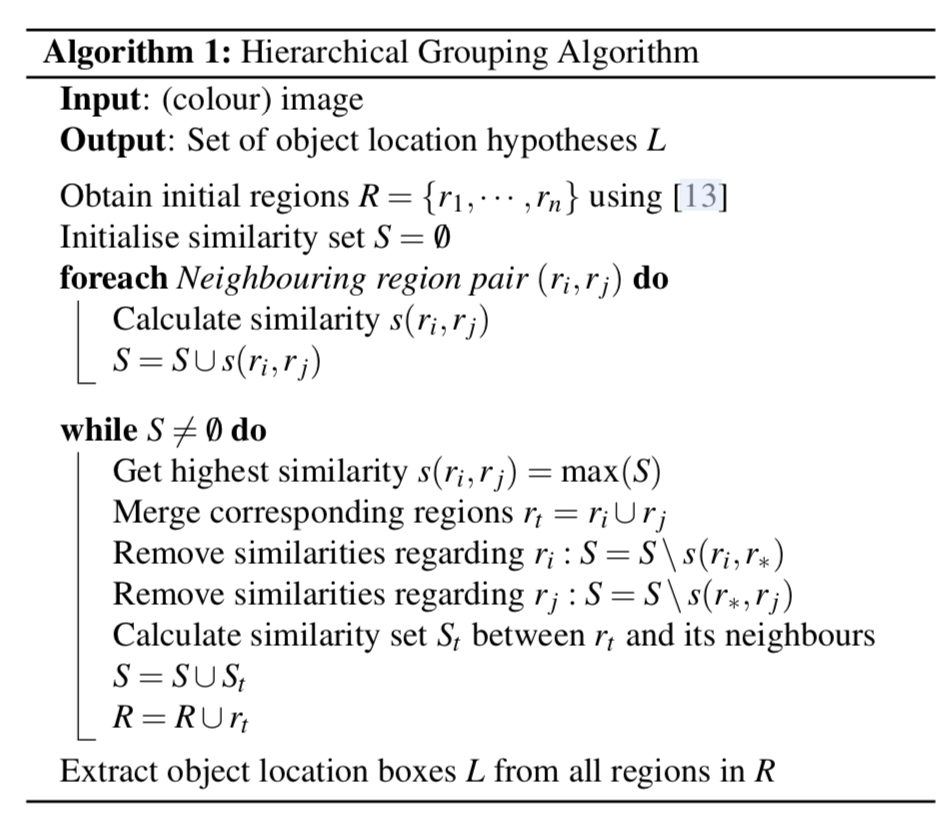## 介绍

Selective Search for Object Recognition立足点是为目标识别(Object Recognition)产生可能的目标区域。1、使用Efficient Graph-Based Image Segmentation中的图像分割算法生成初始区域$R={r_{1},…,r_{n}}$
2、初始化相似度集合$S=\varnothing$
3、计算两两相邻区域之间的相似度，将其添加到相似度集合S中
4、从相似度集合S中找出相似度最大的两个区域$r_{i}$和$r_{j}$，将其合并成为一个区域$r_{t}$，从相似度集合中除去原先与$r_{i}$和$r_{j}$相邻区域之间计算的相似度，计算$r_{t}$与其相邻区域（原先与$r_{i}$或$r_{j}$相邻的区域）的相似度，将其结果添加的到相似度集合S中。同时将新区域$r_{t}$添加到区域集合R中。
5、获取每个区域的Location，这个结果就是物体位置的可能结果L

## 策略多样化(Diversification Strategies)

### 相似度计算多样性

#### 颜色相似度

$$s_{colour}(r_{i},r_{j})=\sum_{i=1}^{n}min(c^{k}{i},c^{k}{j})$$

$$C_{t} = \frac{size(r_{i})\times C_{i}+size(r_{j})\times C_{j}}{size(r_{i})+size(r_{j})}$$

#### 纹理相似度

$$s_{texture}(r_{i},r_{j})=\sum_{i=1}^{n}ming(t^{k}{i},t^{n}{j})$$

#### 大小相似度

$$s_{size}(r_{i},r_{j}) =1-\frac{size(r_{i})+size(r_{j})}{size(im)}$$

#### 吻合相似度

$$s_{fill}(r_{i},r_{j})=1-\frac{size(BB_{ij})-size(r_{i}-size(r_{j}))}{size(im)}$$

$BB_{ij}$表示$r_{i}和r_{j}$的外接矩形区域。size(im)表示整张图片的像素大小

#### 整体相似度

$$s(r_{i},r_{j}) =a_{1}s_{colour}(r_{i},r_{j})+a_{2}s_{texture}(r_{i},r_{j})+a_{3}s_{size}(r_{i},r_{j})+a_{4}s_{fill}(r_{i},r_{j})$$

## Selective Search用于目标识别

1、先使用HOG或者词袋模型(BoW,Bag-of-Words)
2、训练SVM用于识别目标。

## 总结

Selective Search方式只是得到目标候选区域，并没有对这些目标候选区域的位置进行调整。后续的R-CNN和Fast R-CNN使用Selective Search方式生成目标候选区域，然后使用深度学习的方式对这些候选区域进行识别和位置回归得到更加精确的结果。

## 参考文献

Selective Search for Object Recognition